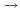## Indicators

•Cited by SciELO
•Access statistics

•Similars in SciELO

## Print version ISSN 1870-3542

#### Abstract

MUNOZ, J.H.  and  QUINTERO, N.. Useful ratios between two-body nonleptonic and semileptonic decays of B mesons. Rev. mex. fís. E [online]. 2011, vol.57, n.1, pp.57-66. ISSN 1870-3542.

We compute important ratios between decay widths of some exclusive two-body nonleptonic and semileptonic B decays, which could be test of factorization hypothesis. We also present a summary of the expressions of the decay widths and differential decay rates of these decays, at tree level, including l = 0 (ground state), l = 1 (orbitally excited) and n = 2 (radially excited) mesons in the final state. From a general point of view, we consider eight transitions, namely HP,V, S, A, A' , T, P (2S), V (2S). Our analysis is carried out assuming factorization hypothesis and using the WSB, ISGW and CLFA quark models.

Keywords : B physics; semileptonic decays; nonleptonic decays.

· abstract in Spanish     · text in English     · English (pdf )All the contents of this journal, except where otherwise noted, is licensed under a Creative Commons Attribution License